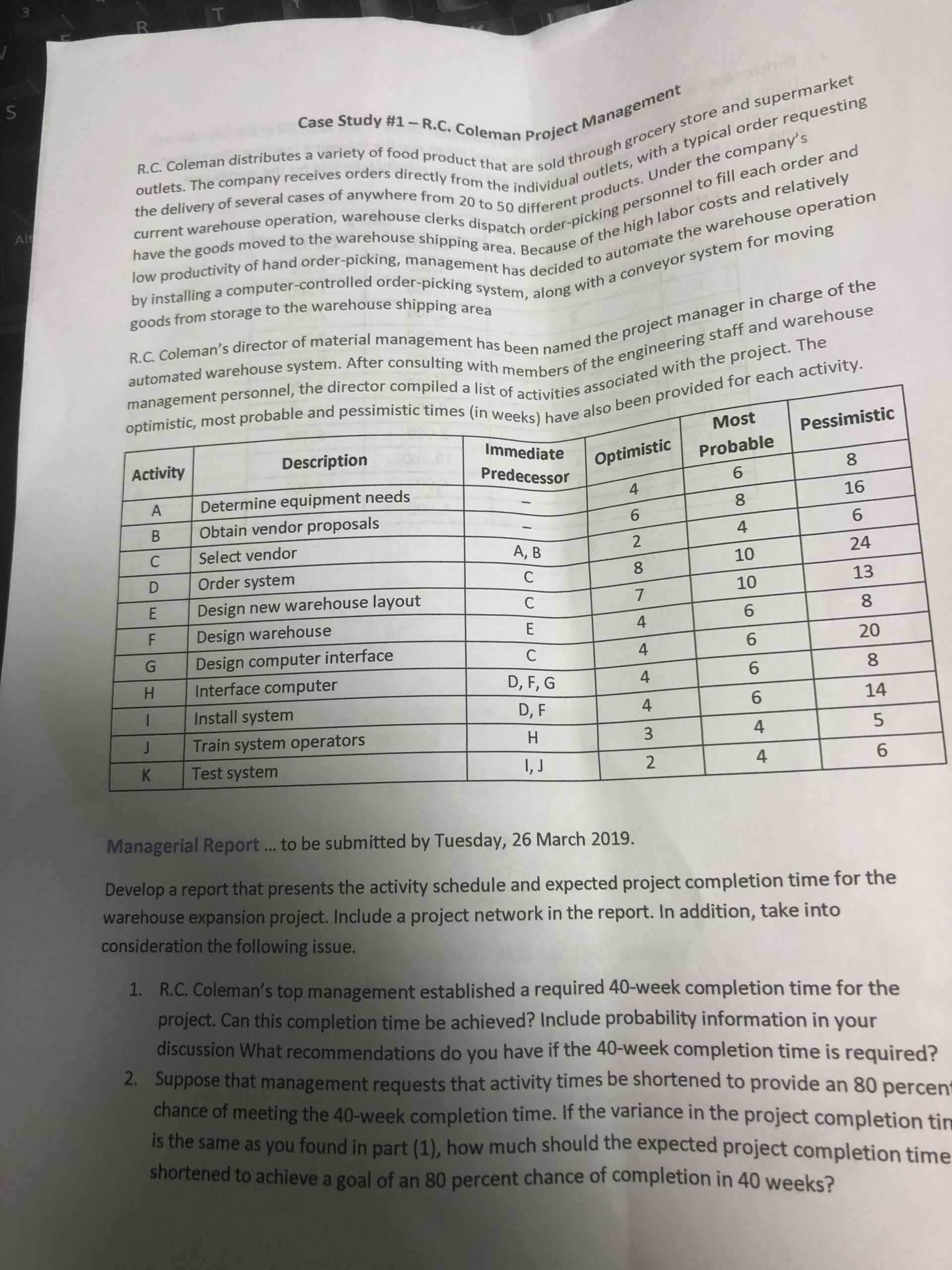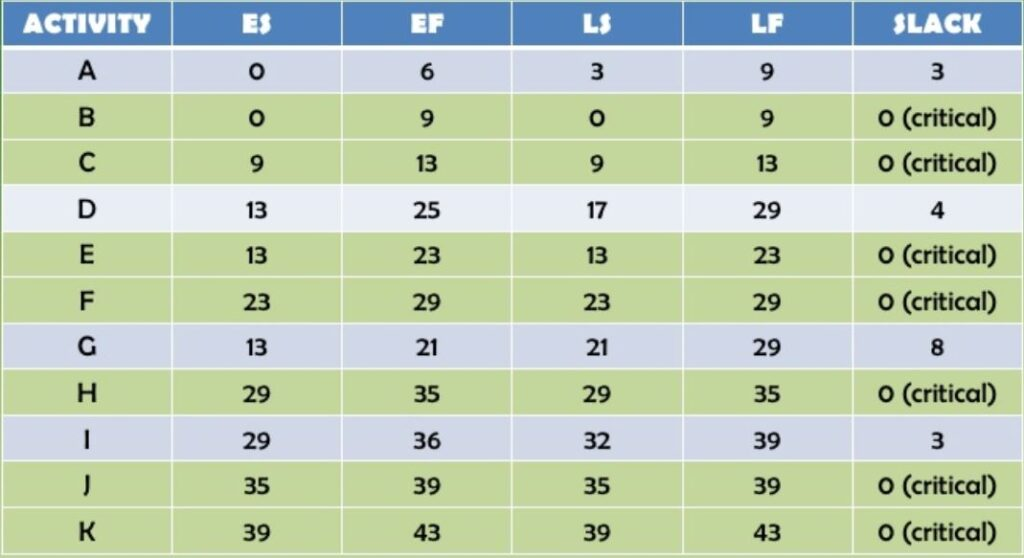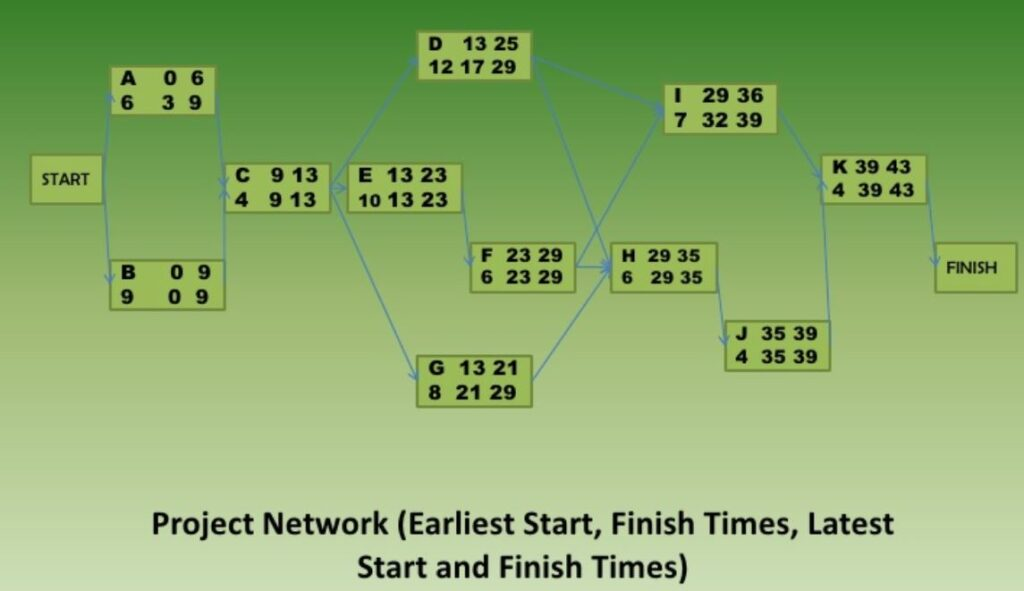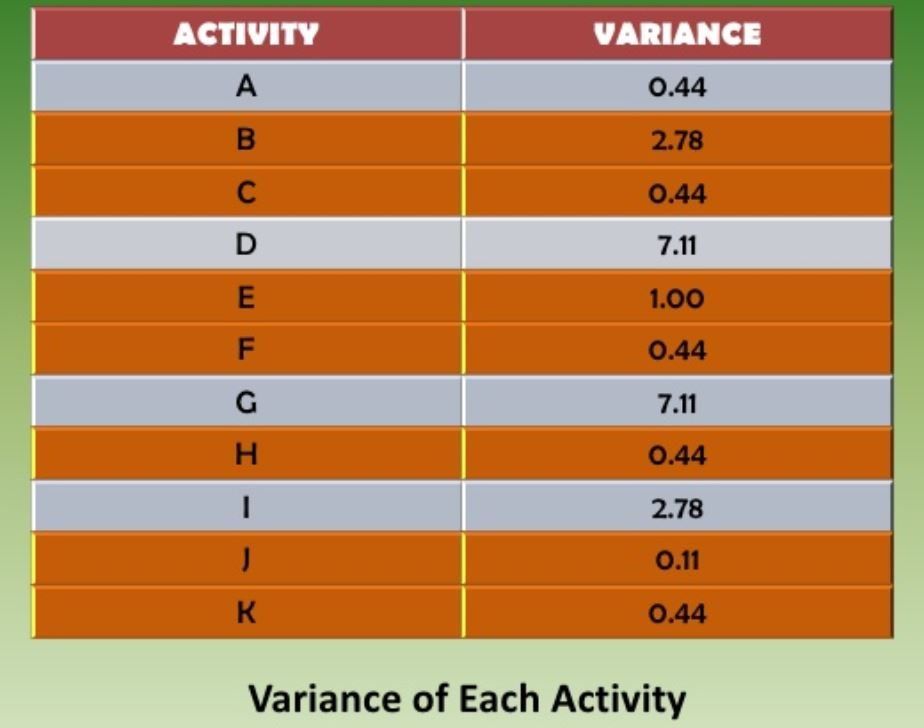# R.C Colemon’s Project Management Solved Assignment Solution Sample

QUESTIONCase Analysis

 Activity Optimistic (to) Most probable (tm) Pessimistic (tp) Expected time= (to+4tm+tp)/6 A 4 6 8 6 B 6 8 16 9 C 2 4 6 4 D 8 10 24 12 E 7 10 13 10 F 4 6 8 6 G 4 6 20 8 H 4 6 8 6 I 4 6 14 7 J 3 4 5 4 K 2 4 6 4Critical Path is having slack value 0

So, the critical path = B-C-E-F-H-J-K

Project completion time = 9+4+10+6+6+4+4= 43 days

The required project completion time of 40 cannot be achieved by the critical path

Variance of each activityVariance of the project completion time

Standard deviation

Probability that the project will be completed within 40 days

Pr(t<=40)

Z=

From normal distribution table

Pr(t<=40)= 0.103= 10.38%

So, there is 10.38% chance that project will be completed by 40 days

b) Probability = 80%

Z value for 0.8 probability = 0.84

0.84= (40-X)/2.38

X= 38

It shows that the project has to be shortened to 38 weeks to achieve the goal of completion of 80% chance.